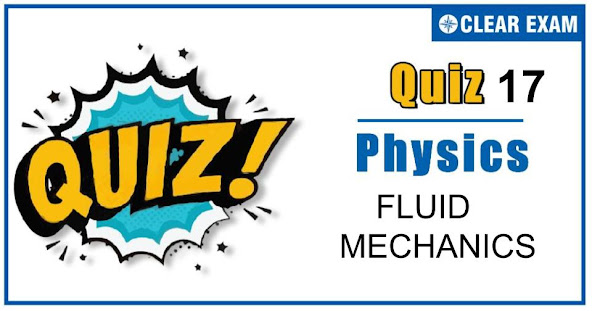## FLUID MECHANICS QUIZ-17

As per analysis for previous years, it has been observed that students preparing for NEET find Physics out of all the sections to be complex to handle and the majority of them are not able to comprehend the reason behind it. This problem arises especially because these aspirants appearing for the examination are more inclined to have a keen interest in Biology due to their medical background. Furthermore, sections such as Physics are dominantly based on theories, laws, numerical in comparison to a section of Biology which is more of fact-based, life sciences, and includes substantial explanations. By using the table given below, you easily and directly access to the topics and respective links of MCQs. Moreover, to make learning smooth and efficient, all the questions come with their supportive solutions to make utilization of time even more productive. Students will be covered for all their studies as the topics are available from basics to even the most advanced. .

Q1. A manometer connected to a close tap reads 3.5×105 Nm(-2). When the valve is opened, the reading of manometer falls to 3.0×105 Nm(-2) , then velocity of flow of water is
•  100 ms(-1)
•  10 ms(-1)
•  1 ms(-1)
•  10√10 ms(-1)
Solution
Bernoulli’s theorem for unit mass of liquid P/ρ+1/2 v2 constant As the liquid starts flowing , it pressure energy decreases 1/2 v2=(P1-P2)/ρ ⟹ 1/2 v2=(3.5×〖10〗5-3×〖10〗5)/〖10〗3 ⟹ v2=(2×0.5×105)/103 ⟹v2=100 ⟹v=10 ms(-1)

Q2.The volume of an air bubble becomes three times as it rises from the bottom of a lake to its surface. Assuming atmospheric pressure to be 75 cm of Hg and the density of water to be 1/10 of the density of mercury, the depth of the lake is
•  5m
•  10m
•  15m
•  20m
Solution
P1 V1=P2 V2⇒(P0+hρg)V=P0×3V ⇒hρg=2P0⇒h=(2×75×13.6×g)/(13.6/10×g)=15 m

Q3.  A uniform rod of density ρ is placed in a wide tank containing a liquid σ(σ>ρ). The depth of liquid in the tank is half the length of the rod. The rod is in equilibrium, with its lower end resting on the bottom of the tank. In this position, the rod makes an angle θ with the horizontal. Then sin⁡θ is equal to
•   1/2 √(σ/ρ)
•  1/2 σ/ρ
•  √(ρ/σ)
•  √(σ/ρ)
Solution
AB=L,AC=L/2;AD=l (say) Let A = area of cross-section of the rod Weight of the rod =AL ρ g acting vertically downwards at C

Q4. Two soap bubbles of radii r1 and r2 equal to 4 cm and 5 cm respectively are touching each other over a common surface AB (shown in figure). Its radius will be
•  4cm
•  4.5cm
•  5cm
•  20cm
Solution
4S/r1 -4S/r2 =4S/r Or 1/r=1/r1 -1/r2 =1/4-1/5=1/20 or r=20 cm

Q5.A 10 cm3 cube floats in water with a height of 4 cm3 remaining above the surface. The density of the material form which the cube is made is
•  0.6 g cm(-3)
•  1.0 g cm(-3)
•  0.4 g cm(-3)
•  0.24 g cm(-3)
Solution
(Volume of cube submerged )/(Total volume)=(Density of cube material)/(Density of water) ⟹ (10-4)/10=d/1 ∴ d=6/10=0.6 g cm(-3)

Q6. A sphere liquid drop of radius R is divided into eight equal to droplets. If surface tension is T, then the work done in this process will be
•  2πR2 T
•  3πR2 T
• 4πR2 T
•  2πRT2
Solution
Volume =4/3 π R3=8×4/3 π r3 or r=R/2 Work done =T×(4 πr2×8-4πR2) =T×4π(R2/4×8-R2 )=4πR2 T

Q7.If a liquid is placed in a vertical cylindrical vessel and the vessel is rotated about its axis, the liquid will take the shape of figure
Solution
For the given angular velocity of rotation, the centrifugal force F∝r; therefore, more liquid will be accumulated near the wall of tube and the liquid meniscus will become concave upwards

Q8.The neck and bottom of a bottle are 3 cm and 15 cm in radius respectively. If the cork is pressed with a force 12 N in the neck of the bottle, then force exerted on the bottom of the bottle is
•  30 N
•  T150N
•  300N
•  600N
Solution
Pressure at neck of bottle p1=F1/A1 =F1/(π r12 ) Similarly, pressure at bottom of bottle p2=F2/A2 =F2/(π r22 ) According to Pascal’s law, liquids transmits pressure equal in all directions. ∴ F2/A2 =F2/(π r22 ) or F2=F1×(r2/r1 )2 =12×(15/3)2=12×25=300N

Q9.A piston of cross-section area 100 cm2 is used in a hydraulic press to exert a force of 107 dynes on the water. The cross-sectional area of the other piston which supports an object having a mass 2000 kg. is
•  100 cm2
•  109 cm2
•  2×104 cm2
•  2×1010 cm2
Solution
P1=P2⇒F1/A1 =F2/A2 ⇒107/102 =(2000×〖10〗3×〖10〗3)/A2 ∴A2=2×104 cm2 (g=980≈103 cm/s2)

Q10. A spherical ball is dropped in a long column of viscous liquid. Which of the following graphs represent the variation of (i)gravitational force with time (ii) viscous force with time (iii) net force acting on the ball with time?
•  Q,R,P
•  R,Q,P
•  P,Q,R
• R,P,Q
Solution
Gravitational force remains constant on the falling spherical ball. It is represented by straight lineP. The viscous force (F=6πηrv) increases as the velocity increases with time. Hence, it is represented by curveQ. Net force = gravitational force – viscous force. As viscous force increases, net force decreases and finally becomes zero. Then the body falls with a constant terminal velocity. It is thus represented by curve R## Want to know more

Please fill in the details below:

## Latest NEET Articles\$type=three\$c=3\$author=hide\$comment=hide\$rm=hide\$date=hide\$snippet=hide

Name

ltr
item
BEST NEET COACHING CENTER | BEST IIT JEE COACHING INSTITUTE | BEST NEET & IIT JEE COACHING: FLUID MECHANICS QUIZ-17
FLUID MECHANICS QUIZ-17